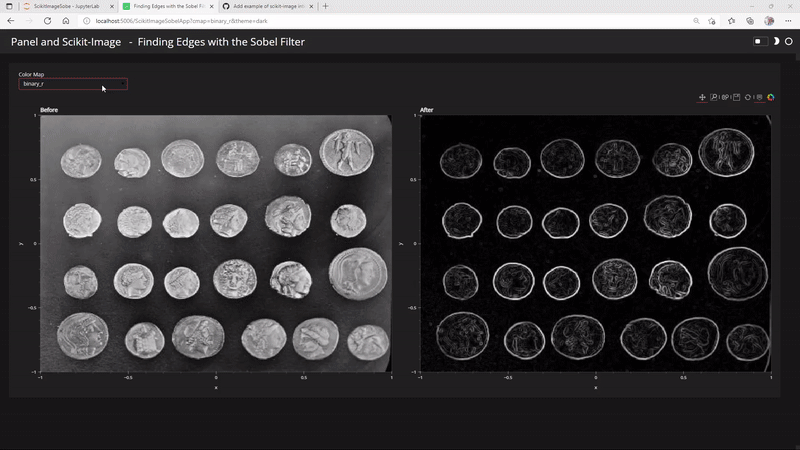# Why does the 2nd image in my layout not show?

I’m trying to create an example of using Panel and HoloViews for the scikit-image docs.

I would like to layout two images next to each other. But the second image just blanks out.

``````import holoviews as hv
import panel as pn
from skimage import data, filters

pn.extension(sizing_mode="stretch_width")

image = data.coins()
edges = filters.sobel(image)

bounds = (-1, -1, 1, 1)
height, width = image.shape

before_img = hv.Image(image, bounds=bounds).opts(
cmap="binary_r", height=height, width=width, title="Before", active_tools=["box_zoom"]
)
after_img = hv.Image(edges, bounds=bounds).opts(
cmap="binary_r", height=height, width=width, title="After", active_tools=["box_zoom"]
)

component = pn.Row(before_img+after_img).servable()
``````

I would expect the 2nd image to look like

It is because the colormap is synced, try `(before_img+after_img).opts(shared_axes=False)`. If you add colorbar to you example it gets more clear:

If you want to be able to move the plots at the same time you could multiply edges with 255, this should properly be seen as a little hack.

1 Like

Even better is to just set the vdims for the two Images. Like:

``````before_img = hv.Image(image, bounds=bounds, vdims=("image")).opts(
cmap="binary_r",height=height, width=width, title="Before", active_tools=["box_zoom"], colorbar=True
)
after_img = hv.Image(edges, bounds=bounds, vdims=("edges")).opts(
cmap="binary_r",height=height, width=width, title="After", active_tools=["box_zoom"], colorbar=True
)

(before_img+after_img)
``````

1 Like

Ahhhh. Thanks @Hoxbro

1 Like

It’s now are Feature Request for the docs in Scikit-image Add example of scikit-image interactive tool build using Panel and HoloViews to the docs · Issue #6023 · scikit-image/scikit-image (github.com). You can find the code/ notebook there.There is video walkthrough here scikit image panel holoviews - YouTube

1 Like